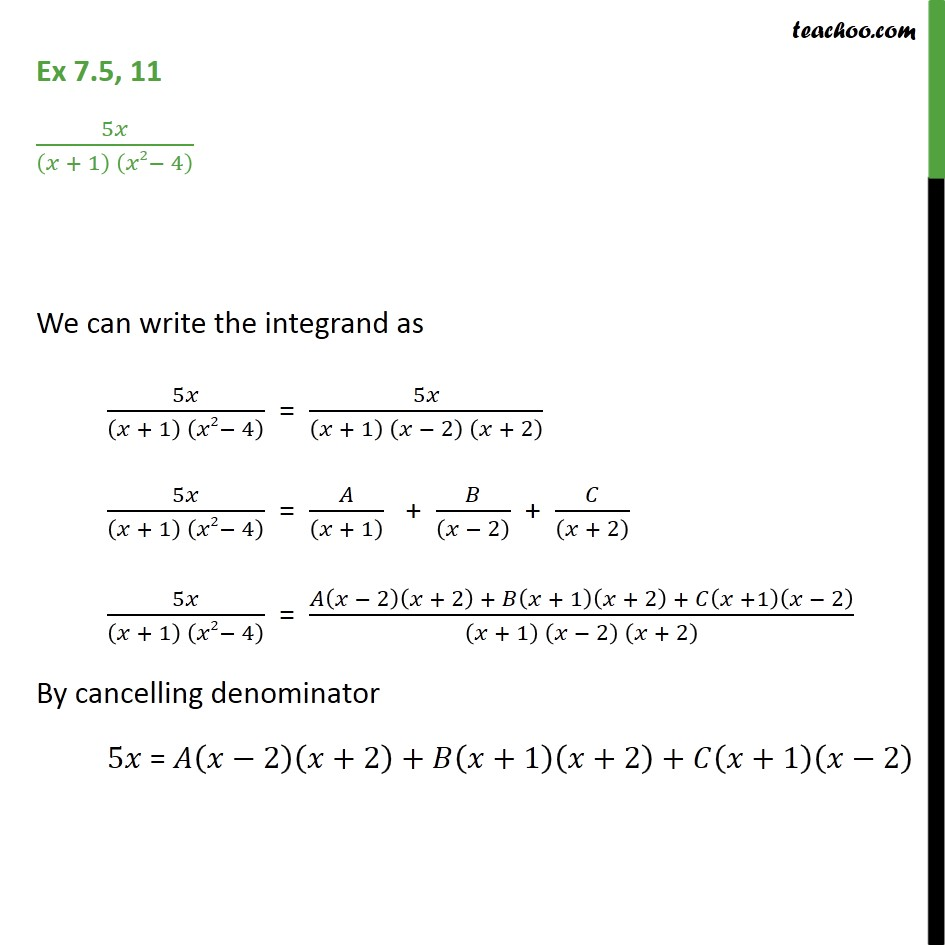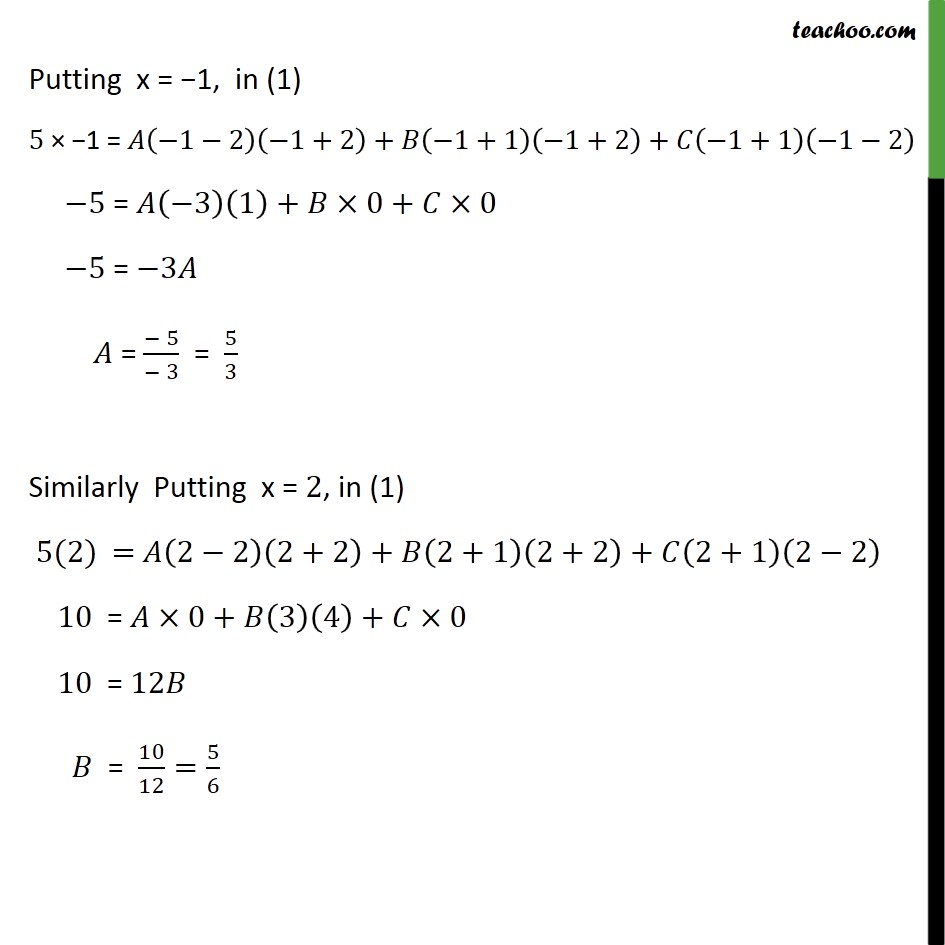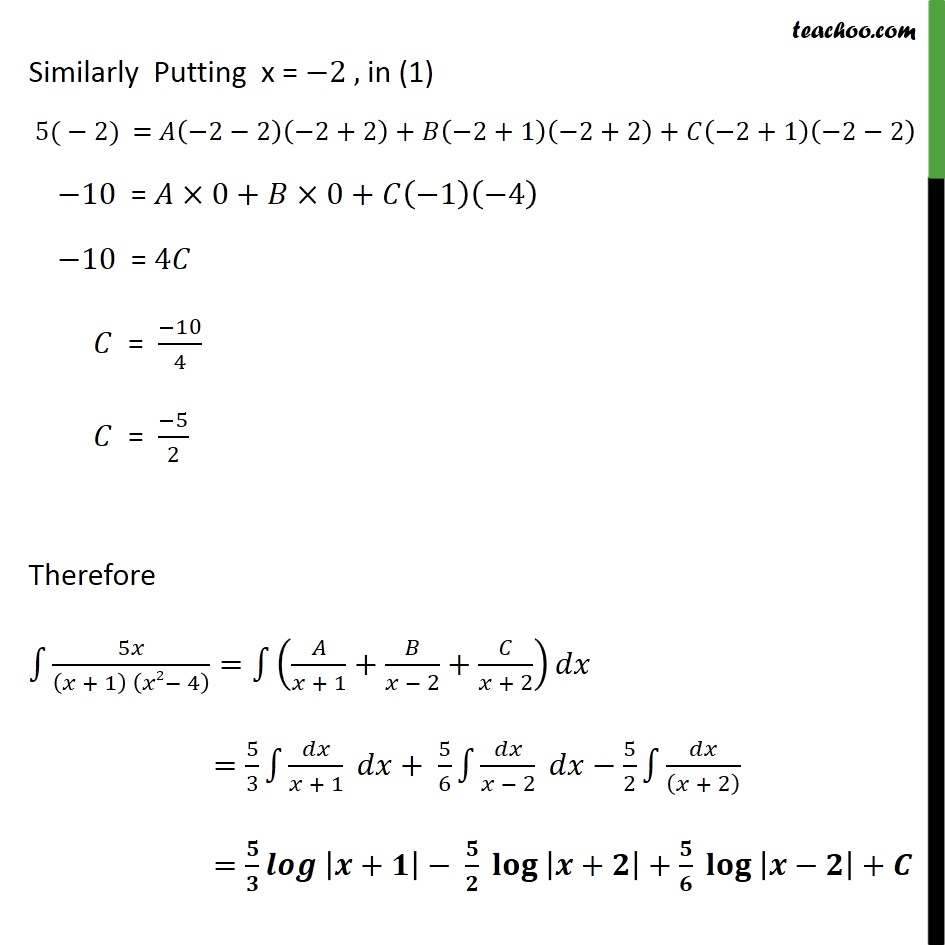1. Chapter 7 Class 12 Integrals
2. Serial order wise
3. Ex 7.5

Transcript

Ex 7.5, 11 5𝑥﷮ 𝑥 + 1﷯ 𝑥2− 4﷯﷯ We can write the integrand as 5𝑥﷮ 𝑥 + 1﷯ 𝑥2− 4﷯﷯ = 5𝑥﷮ 𝑥 + 1﷯ 𝑥 − 2﷯ 𝑥 + 2﷯﷯ 5𝑥﷮ 𝑥 + 1﷯ 𝑥2− 4﷯﷯ = 𝐴﷮ 𝑥 + 1﷯﷯ + 𝐵﷮ 𝑥 − 2﷯﷯ + 𝐶﷮ 𝑥 + 2﷯﷯ 5𝑥﷮ 𝑥 + 1﷯ 𝑥2− 4﷯﷯ = 𝐴 𝑥 − 2﷯ 𝑥 + 2﷯ + 𝐵 𝑥 + 1﷯ 𝑥 + 2﷯ + 𝐶 𝑥 +1﷯ 𝑥 − 2﷯﷮ 𝑥 + 1﷯ 𝑥 − 2﷯ 𝑥 + 2﷯﷯ By cancelling denominator 5𝑥 = 𝐴 𝑥−2﷯ 𝑥+2﷯+𝐵 𝑥+1﷯ 𝑥+2﷯+𝐶 𝑥+1﷯ 𝑥−2﷯ Putting x = −1, in (1) 5 × −1 = 𝐴 −1−2﷯ −1+2﷯+𝐵 −1+1﷯ −1+2﷯+𝐶 −1+1﷯ −1−2﷯ −5 = 𝐴 −3﷯ 1﷯+𝐵×0+𝐶×0 −5 = −3𝐴 𝐴 = − 5﷮− 3﷯ = 5﷮3﷯ Similarly Putting x = 2, in (1) 5(2) = 𝐴 2−2﷯ 2+2﷯+𝐵 2+1﷯ 2+2﷯+𝐶 2+1﷯ 2−2﷯ 10 = 𝐴×0+𝐵 3﷯ 4﷯+𝐶×0 10 = 12𝐵 𝐵 = 10﷮12﷯= 5﷮6﷯ Similarly Putting x = −2 , in (1) 5(−2) = 𝐴 −2−2﷯ −2+2﷯+𝐵 −2+1﷯ −2+2﷯+𝐶 −2+1﷯ −2−2﷯ −10 = 𝐴×0+𝐵×0+𝐶 −1﷯ −4﷯ −10 = 4𝐶 𝐶 = −10﷮4﷯ 𝐶 = −5﷮2﷯ Therefore ﷮﷮ 5𝑥﷮ 𝑥 + 1﷯ 𝑥2− 4﷯﷯﷯= ﷮﷮ 𝐴﷮𝑥 + 1﷯+ 𝐵﷮𝑥 − 2﷯+ 𝐶﷮𝑥 + 2﷯﷯﷯𝑑𝑥 = 5﷮3﷯ ﷮﷮ 𝑑𝑥﷮𝑥 + 1﷯﷯ 𝑑𝑥+ 5﷮6﷯ ﷮﷮ 𝑑𝑥﷮𝑥 − 2﷯﷯ 𝑑𝑥− 5﷮2﷯ ﷮﷮ 𝑑𝑥﷮ 𝑥 + 2﷯﷯﷯ = 𝟓﷮𝟑﷯ 𝒍𝒐𝒈 ﷮ 𝒙+𝟏﷯﷯− 𝟓﷮𝟐﷯ 𝐥𝐨𝐠 ﷮ 𝒙+𝟐﷯﷯+ 𝟓﷮𝟔﷯ 𝐥𝐨𝐠 ﷮ 𝒙−𝟐﷯﷯+𝑪

Ex 7.5

Chapter 7 Class 12 Integrals
Serial order wise